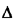## 3.5.2 Summary of Method

We now summarize Crofton's method for computing mean values as follows:

There are N points independently and uniformly distributed over some region R. One wants to calculate the mean value of some function that depends on the relative position of the points. Expand the space by a small incrementR, while preserving the same geometric shape of the region. Now distribute the point positions uniformly and independently over the expanded region R +R. One can then relate the desired mean value to the mean of the same function, conditioned on the event that one of the points lies within the small increment. This conditional mean value is usually easier to calculate than is the desired mean value. The expansion process yields a (usually) simple first-order differential equation whose solution is the desired mean value. It is important to note that the form of the differential equation depends only on R, N, and the uniform probability laws of the point positions; the solution depends on the specific form of the function whose mean value is desired.

Problems 3.18-3.21 ask you to apply Crofton's method in certain urban applications.